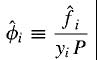# Fugacity coefficient of an ideal solution

Hi all. I have some confusion regarding the fugacity coefficient of a species in an ideal solution so hoping someone could clear it up for me. According to my textbook, for fugacity coefficient of a species i in a solution:So if we were talking about an ideal liquid mixture of let's say species A and B, then the denominator yi should be replaced by xi as per stated by the textbook. So if i were to calculate the fugacity coefficient of species A, my question is what is the physical significance of xAP? is it the vapour pressure of the vapour of species A above the liquid components? Why is it calculated in such a way (seems like the same way as how partial pressure of gas mixture is calculated). From what i know, the calculation of vapour pressure of a species in ideal mixture should be xiPo where Po is the vapour pressures of i if it were to exist as pure liquid